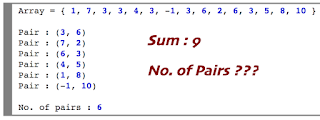Showing posts with label Pair of given sum. Show all posts
Showing posts with label Pair of given sum. Show all posts## How to find integer pairs with given sum

Given an array of integers, and a number ‘sum’, find the number of pairs of integer in the array whose sum is equal to ‘sum’.

Array can be a combination of +ve, -ve and duplicate elements. Where we need to get the list of all unique pairs from the given array which makes sum is equal to given number. Easy solution with O(N) time complexity will be
• Iterate each element from array and store it in Set.
• Compare the elements difference from sum as whether present in the Set or not. If present then print the pair with element and sum difference.
• Remove the difference element from Set to avoid generating duplicate pair.
Lets see simple Java solution

```import java.util.HashSet;

public class PairSum {

public static void main(String[] args) {

int[] val = new int[] { 1, 7, 3, 3, 3, 4, 3, -1, 3, 6, 6, 6, 6, 2, 6, 3, 3, 5, 8, 10 };
PairSum obj = new PairSum();
obj.getPairs(val, 9);
}

public void getPairs(int[] val, int sum) {

HashSet<Integer> set = new HashSet<Integer>();
int count = 0;
for (int i : val) {
if (set.contains(sum - i)) {
System.out.println("Pair : (" + (sum - i) + ", " + i + ")");
set.remove(sum - i); // Removing existing value from set to avoid duplicate pair to print
count++;
}
}

System.out.println("\nNo. of pairs : "+count);
}
}
```

OUTPUT:

```Pair : (3, 6)
Pair : (7, 2)
Pair : (6, 3)
Pair : (4, 5)
Pair : (1, 8)
Pair : (-1, 10)

No. of pairs : 6
```